# HIQL: Offline Goal-Conditioned RL with Latent States as Actions NeurIPS 2023

## Abstract

Unsupervised pre-training has recently become the bedrock for computer vision and natural language processing. In reinforcement learning (RL), goal-conditioned RL can potentially provide an analogous self-supervised approach for making use of large quantities of unlabeled (reward-free) data. However, building effective algorithms for goal-conditioned RL that can learn directly from diverse offline data is challenging, because it is hard to accurately estimate the exact value function for faraway goals. Nonetheless, goal-reaching problems exhibit structure, such that reaching distant goals entails first passing through closer subgoals. This structure can be very useful, as assessing the quality of actions for nearby goals is typically easier than for more distant goals. Based on this idea, we propose a hierarchical algorithm for goal-conditioned RL from offline data. Using one action-free value function, we learn two policies that allow us to exploit this structure: a high-level policy that treats states as actions and predicts (a latent representation of) a subgoal and a low-level policy that predicts the action for reaching this subgoal. Through analysis and didactic examples, we show how this hierarchical decomposition makes our method robust to noise in the estimated value function. We then apply our method to offline goal-reaching benchmarks, showing that our method can solve long-horizon tasks that stymie prior methods, can scale to high-dimensional image observations, and can readily make use of action-free data.

## Why is Offline Goal-Conditioned RL Hard?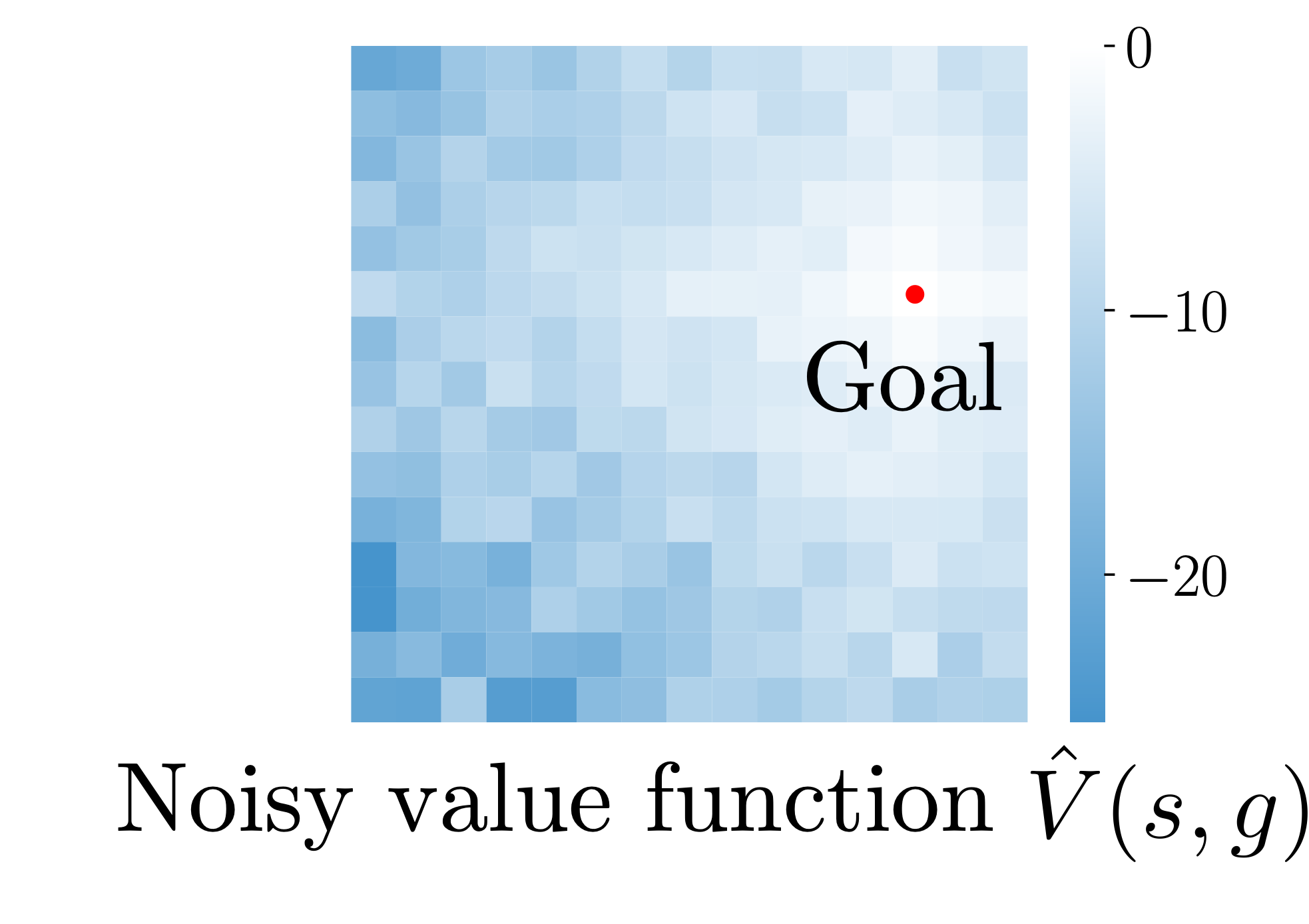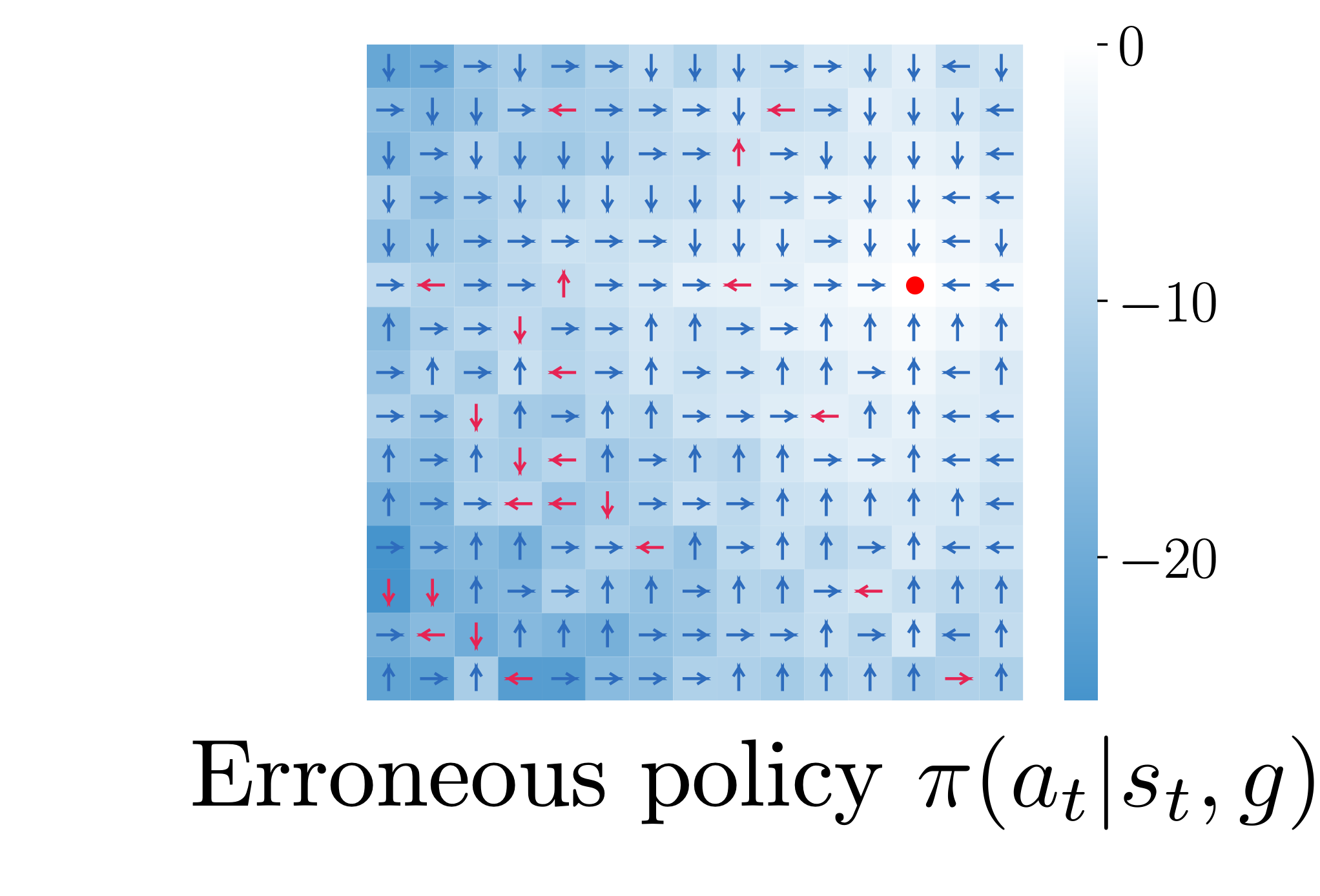• Offline goal-conditioned RL is challenging because the "signal-to-noise" ratio of the learned value function can be very low for faraway goals.
• As illustrated in the figure above, as a state gets farther from the goal, the policy becomes more erroneous due to the noise in the learned value function.

## Solution: Hierarchical Policy Extraction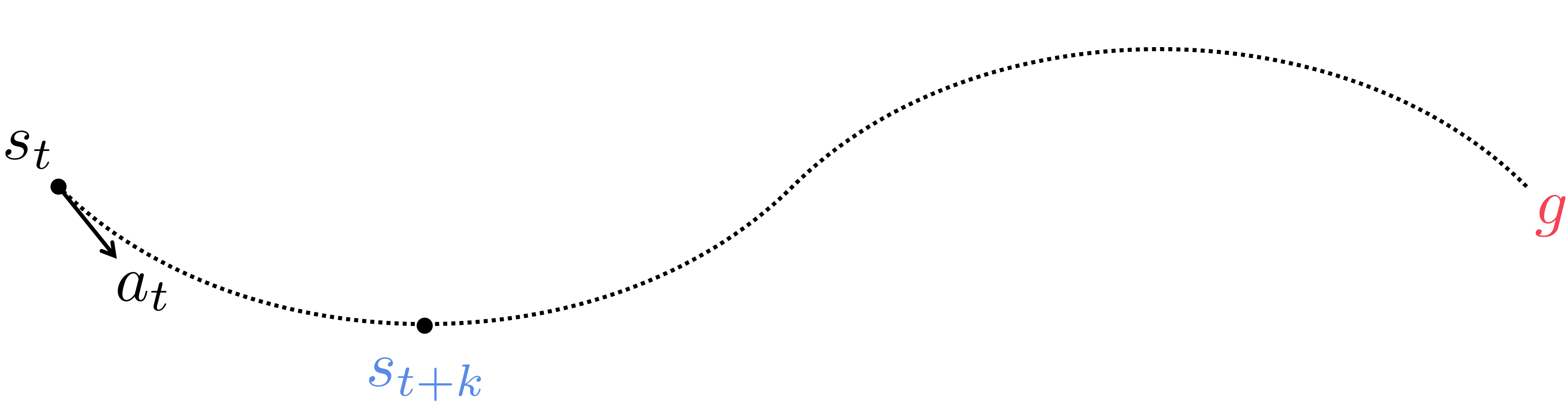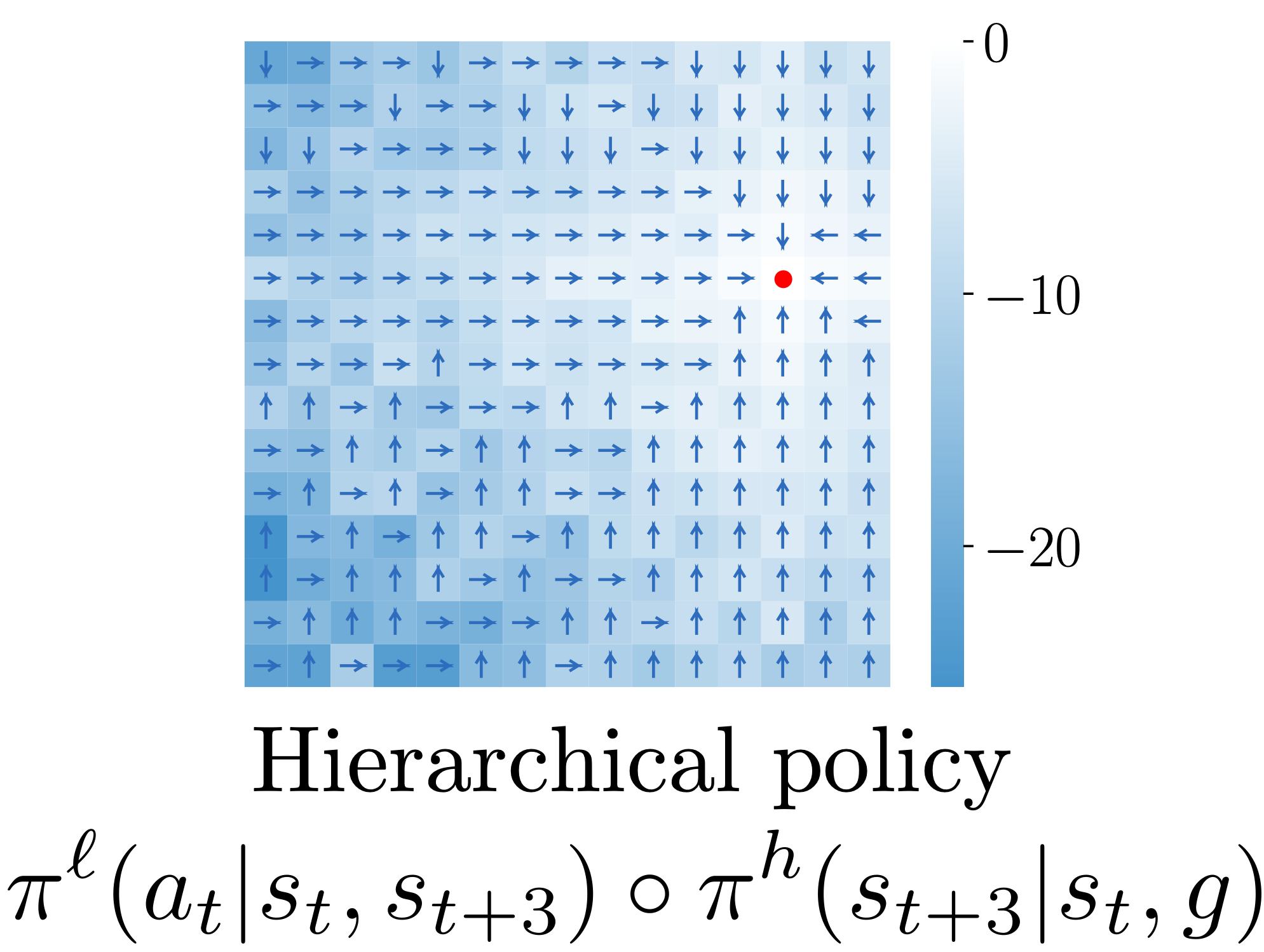• To address this issue, we extract hierarchical policies from the same value function.
• The high-level policy $$\pi^h(s_{t+k}|s_t, g)$$, which treats states as actions, produces an intermediate subgoal $$s_{t+k}$$ to reach the goal $$g$$.
• The low-level policy $$\pi^\ell(a_t|s_t, s_{t+k})$$ produces actions to reach the subgoal.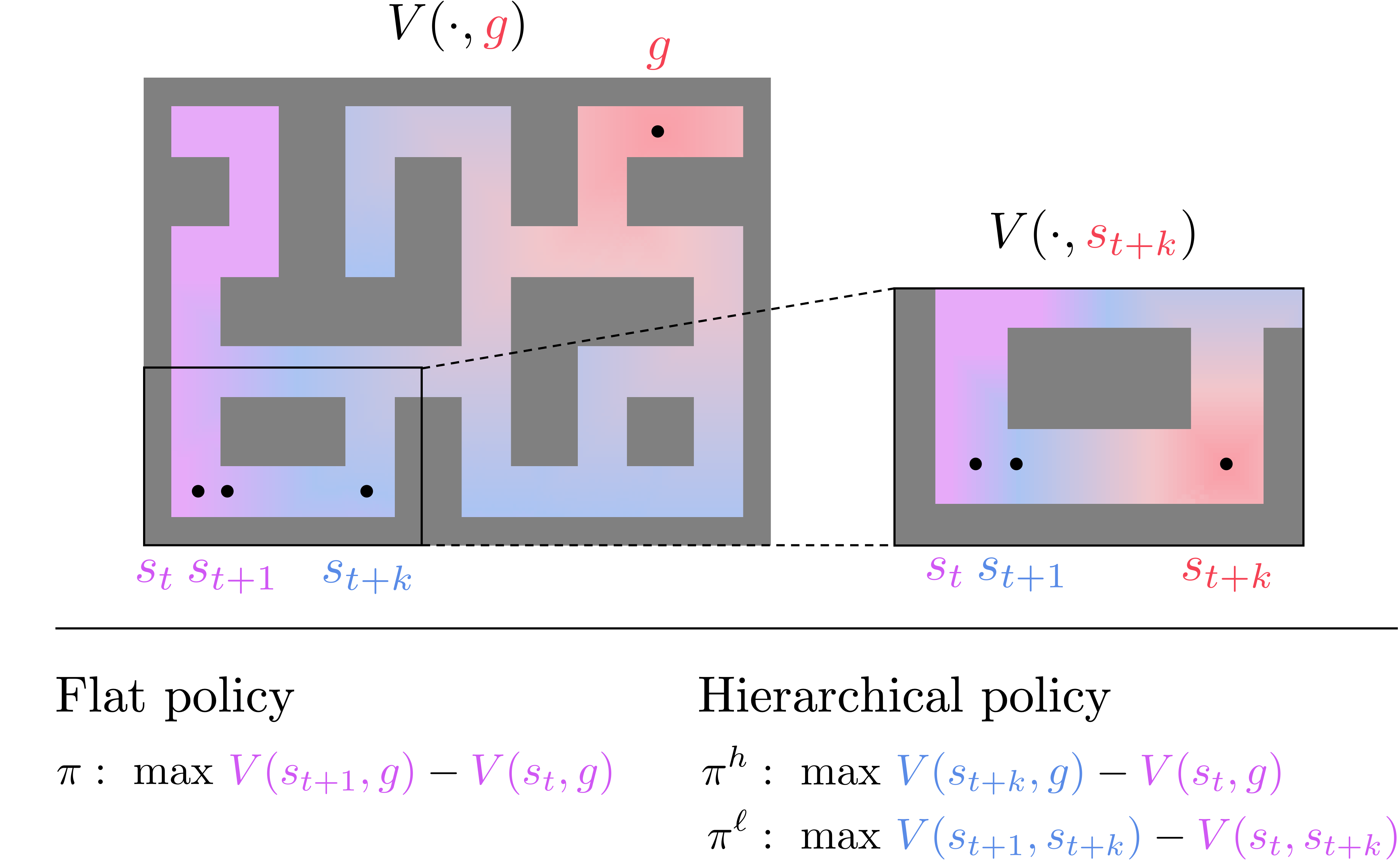• This hierarchical structure improves the "signal-to-noise" ratio due to the improved relative differences between values, even though both policies are extracted from the same value function.

## Hierarchical Implicit Q-Learning (HIQL)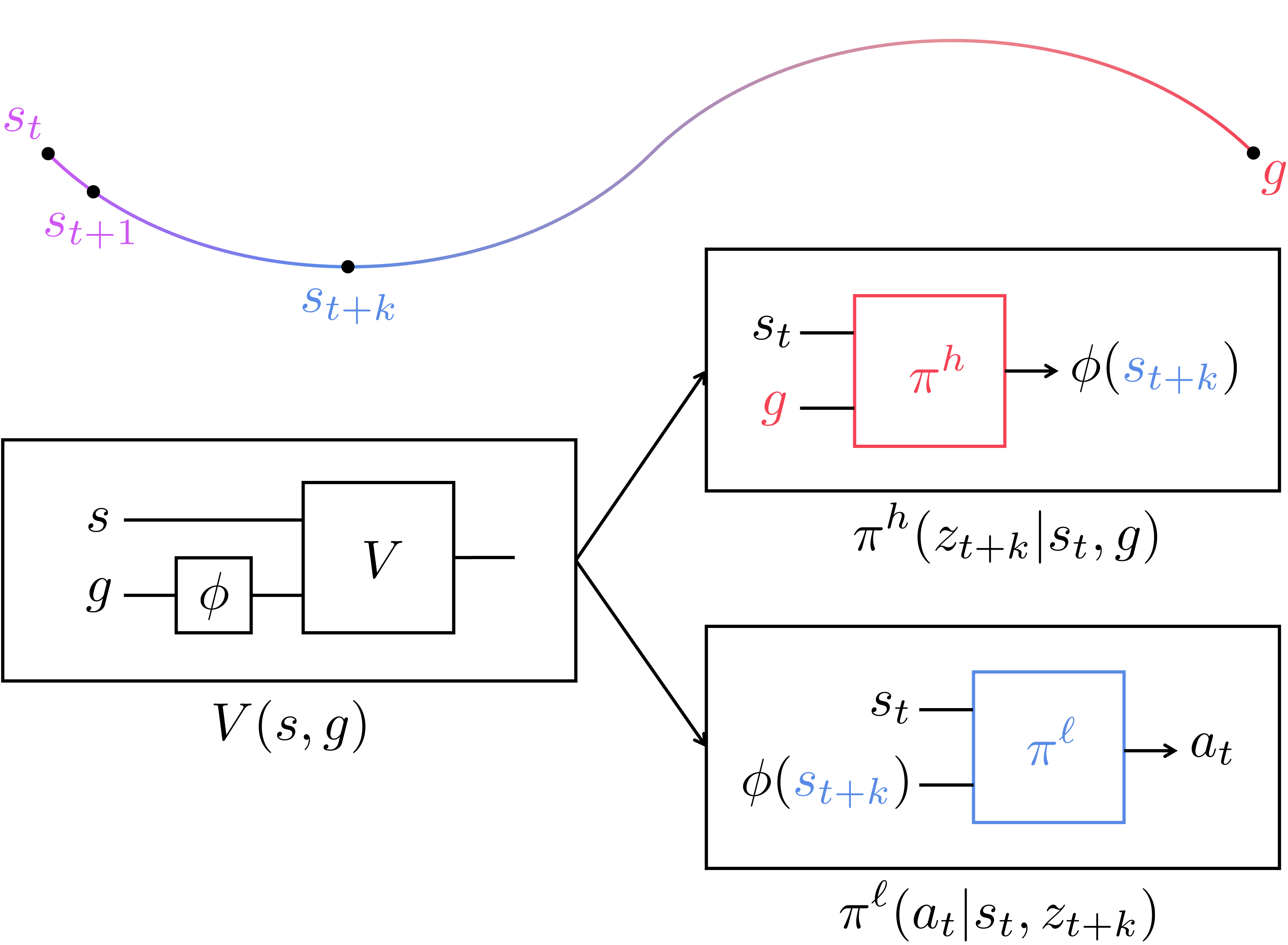### HIQL Objective

• Based on the idea above, our method, HIQL, trains a single goal-conditioned value function with IQL, from which we extract two policies with AWR.
• The value function minimizes the IQL loss: $$\mathbb{E}[L_2^\tau (r(s, g) + \gamma \bar{V}(s', g) - V(s, g))]$$, where $$L_2^\tau$$ is the expectile loss.
• The high-level policy maximizes the high-level AWR objective: $$\mathbb{E}[\log \pi^h(s_{t+k} \mid s_t, g) e^{\beta (V(s_{t+k}, g) - V(s_t, g))}]$$.
• The low-level policy maximizes the low-level AWR objective: $$\mathbb{E}[\log \pi^\ell(a_t \mid s_t, s_{t+k}) e^{\beta (V(s_{t+1}, s_{t+k}) - V(s_t, s_{t+k}))}]$$.

### Representations for Subgoals

• Since directly predicting high-dimensional subgoals is challenging, our high-level policy produces low-dimensional representations of subgoals.
• To do this, HIQL parameterizes the value function as $$V(s, \phi(g))$$ and simply uses $$\phi(g)$$ as the goal representation. We prove that this simple value-based representation does not lose any information about the goal for the policy.
• Our high-level policy hence produces $$z_{t+k} = \phi(s_{t+k})$$ instead of $$s_{t+k}$$.

### Leveraging Action-Free Data

• One important property of HIQL is that only the low-level policy requires action labels.
• As such, we can leverage a potentially large amount of video data, when (pre-)training the value function and high-level policy.

## Environments## Results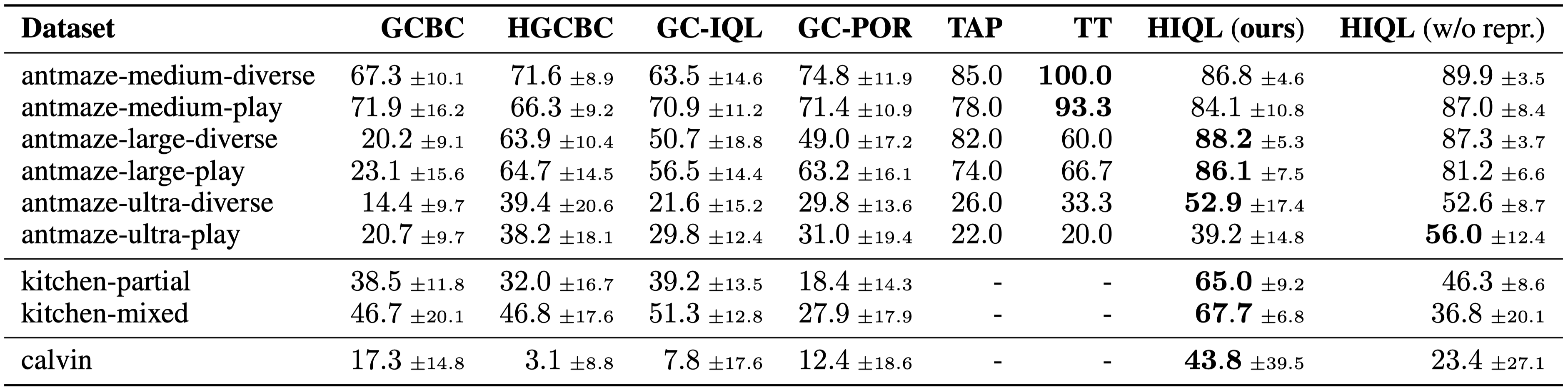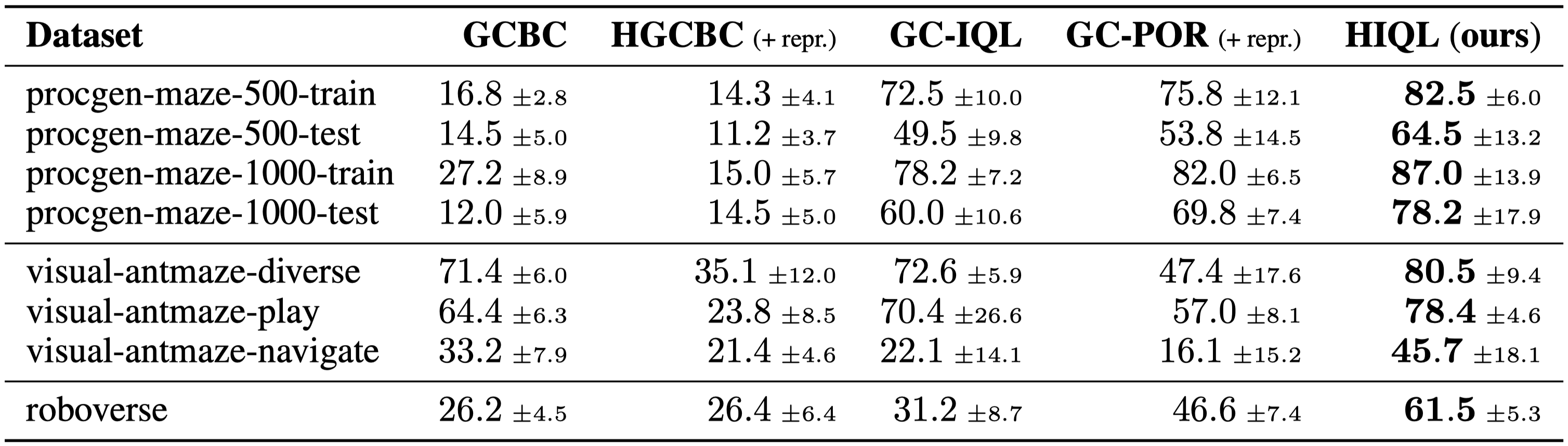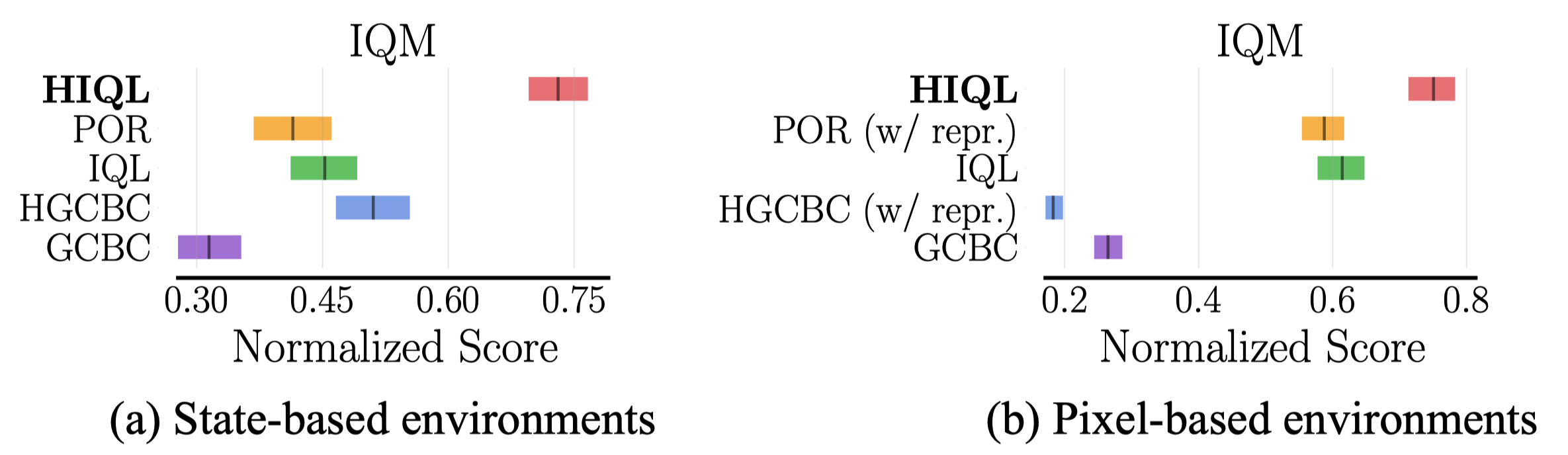• HIQL mostly achieves the best performance in both state-based and pixel-based benchmarks.

## Procgen Maze Videos

• To visualize subgoals in the latent representation space, we find the maze positions that have the closest representations to the outputs of the high-level policy.

The website template was borrowed from Michaël Gharbi and Jon Barron.